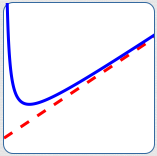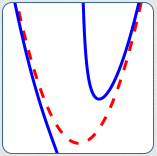﻿ Introduction to Asymptotes

#Introduction to Asymptotes

DEFINITION asymptote
An ‘asymptote’ (pronounced AS-sim-tote) is a curve (usually a line) that another curve gets arbitrarily close to as $\,x\,$ approaches $\,+\infty\,,$ $\,-\infty\,,$ or a finite number.

Rational functions usually have interesting asymptote behavior.

Asymptotes exhibited by rational functions come in different flavors, as shown below:horizontal asymptote

The dashed red line is horizontal.

The blue curve is getting closer and closer to this horizontal red line as $\,x\rightarrow\infty\,$ and as $\,x\rightarrow -\infty\,.$

Thus, the red line is
a horizontal asymptote.vertical asymptote

The dashed red line is vertical.

The blue curve is getting closer and closer to this vertical red line as $\,x\,$ approaches a finite number (from the right, and from the left).

Thus, the red line is a vertical asymptote.slant asymptote

The dashed red line is not horizontal, and not vertical. It is ‘slanted’.

The blue curve is getting closer and closer to this ‘slanted’ red line as $\,x\rightarrow\infty\,.$

Thus, the red line is a slant asymptote.asymptotes that are not lines

The dashed red curve is not a line.

The blue curve is getting closer and closer to this red curve as $\,x\rightarrow\infty\,$ and as $\,x\rightarrow -\infty\,.$

Thus, the red curve is an asymptote that is not a line.

Vertical, horizontal and slant asymptotes are studied in more detail in the next few sections.